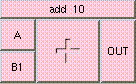ADDTHE BOX'S MAIN HELP: This is the addition function. The result is A + B + .... A and B may be scalar, vectors, or colors. The result will be a scalar if both A and B are scalars. The result will be a vector if either A or B is a vector. The result will be a color if either A or B is a color. A type mis-match will result from the addition of vectors and colors. A (input): This input may be a scalar, vector, or color. This is the value to be added. B (vinput): This input may be a scalar, vector, or color. This is the value to be added. OUT (output): This is the addition function. The result is A + B + .... A and B may be scalar, vectors, or colors. The result will be a scalar if both A and B are scalars. The result will be a vector if either A or B is a vector. The result will be a color if either A or B is a color. A type mis-match will result from the addition of vectors and colors.

<-- BACK TO Math